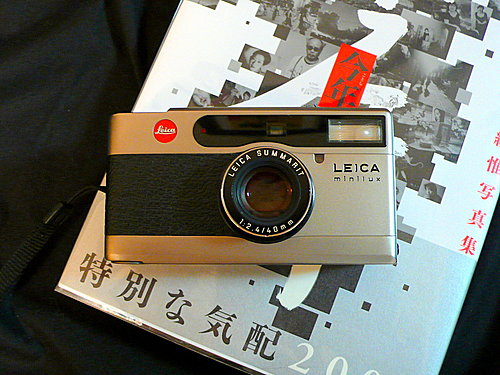Leica Minilux 定位上屬於“高階輕便相機“，機身材質使用鈦金屬、可以手動調整光圈與對焦距離、具有EV補正。很自然的我會把他與我另一部愛機Contax T2 做比較，感覺外型上還是Contax T2 在線條設計上比較有修飾，Leica Minilux 則稍稍方正了點，有點呆板。

Leica Minilux 搭載的是Leica Summarit f2.4 / 40mm 鏡頭，f2.4的大光圈對於習慣街拍不方便用閃燈的攝影者來說自然在應用上更方便。而這顆鏡頭在之後Leica 推出更高階的輕便相機Leica CM 時仍然沿用，可見其品質優異。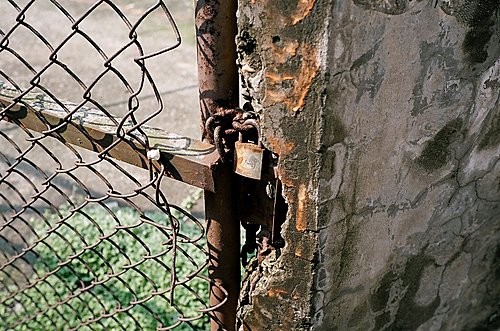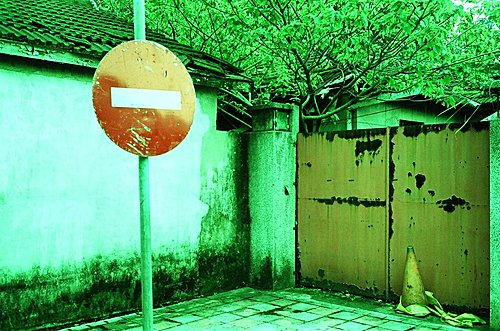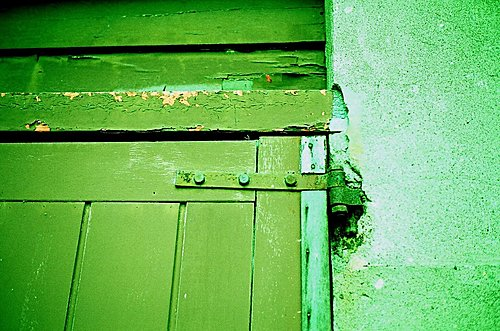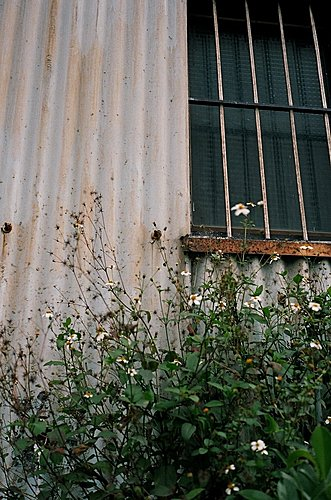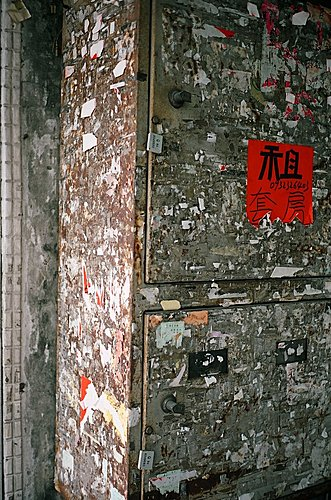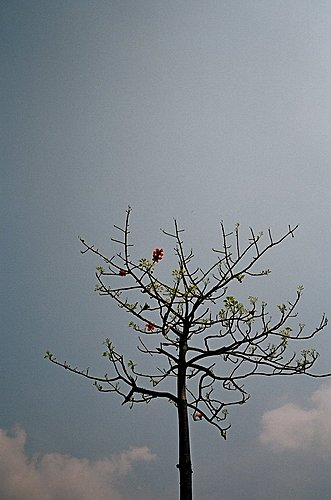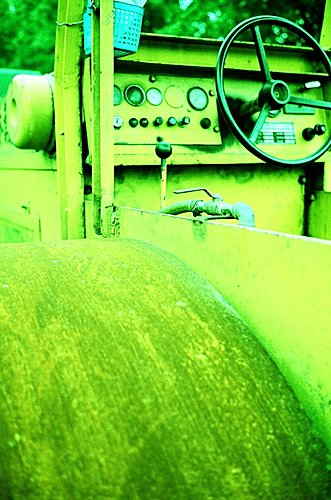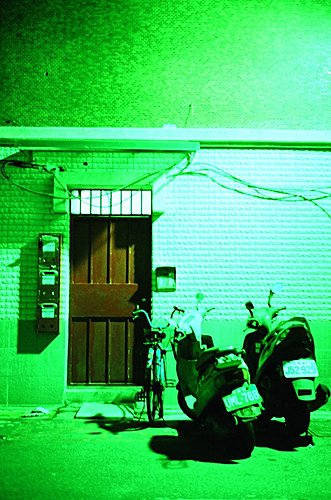===================  廣告時間  ===================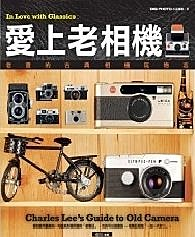#### 89 意見

1.先來搶頭香~ :))

您分享的影像也太毒了吧~
好棒的色調阿 ;;)

我當時在買傻瓜相機的時候也是想買這台說
只不過那個可怕的E02讓我不敢動手
沒有多餘的錢去修阿
所以最後買了一部mju 2
也是挺好玩的呢 :)

1.[miu] 2是部物美價廉的傻瓜相機，我自己也擁有兩部呢 :D

其實老相機真的一切都只能隨緣，E02雖然可怕，不過也就只好想能拍一張是一張了

2.想不到Leica 跟Panasonic的淵源那麼久 :-?

謝謝分享唷^^"

1.是啊，如果我查到的資料正確的話，這兩家公司的合作關係還真的是源遠流長呢 :)

3.第一張也太毒了吧!!
老哥.下次放正片試看看.但不要負沖喔!!
之前我發現我表哥也有一台.目前我正在進行頂讓勸說.

1.手上的這幾捲正片因為是過期（2001到期）的，所以都用來負沖玩玩
之後應該要試試看正片的效果
祝你順利入手Minilux，我這部的入手價是\$7,000，供你參考

4.偏綠的那幾張好 lomo 的感覺喔... ;)

1.是啊，因為是用一般LOMO玩家常玩的正沖負效果 :)

5.提拉米蘇天行者2007年4月23日 下午5:22

原來這台跟Panasonic有很深的關係呀~不過我倒是更驚訝Panasonic的老闆竟然對生產相機有興趣~~(印象中他們專產家電)
不過話說回來，這台Leica Minilux在操作上只要按快門即可嗎?還是他可以玩其他手動功能?

1.Minilux可以手動調整光圈（快門由程式配合光圈決定）與對焦距離，所以在應用上還蠻方便有彈性的

6.贊成路易所說，第一張真的有夠毒!
最近買了批RDP III，希望也有些好照片出現..

1.Minilux如果不是有E02的隱憂，說實在是部還蠻好用的相機，手感扎實，拍出來的照片也不錯

7.接近無限透明的藍2007年4月23日 下午9:23

恭喜囉，不過我還是勸直上，總覺得名人最後還是會買M series的，呵呵。
另外，最近用kodakchrome 64拍了一些，真的不同凡響，反而我覺得我拍的很爛。

1.我之前已經敗了Leica M3了，也Po了一些M3 + 35mm鏡頭的照片...
不過M3測光稍稍不方便，需要外接測光表，以我的拍法來說還是輕便相機（傻瓜機）比較適合我

8.之前考慮購入隨身機時也考慮過minilux,不過EO2問題始終揮之不去,有使用minilux的格有已經修過兩次EO2了.
之前我曾在某站下標購買minilux,雖然最後沒有成交,不過賣家提供一個方法,就是在怪兵器買個鏡頭蓋,不要使用minilux的鏡頭伸縮功能,不拍時使用鏡頭蓋蓋住,等待相機自動休眠就行,這樣鏡頭不要伸縮太頻繁,就可以避免EO2,由於我手上沒有這台機器,所以我沒法這樣試,當然也因為這樣失去了隨身機的效果,所以我也沒買minilux.
這個訊息只能提供大老闆參考. ;) 畢竟這台好像機能多拍久一些都是一種幸福

1.正如Wen大所說，如果鏡頭不收，似乎就失去了隨身機的意義（尤其鏡頭伸出來我還更擔心攜帶時會撞到造成偏移），所以我決定挑戰E02的宿命，就正常拍看看何時會發生。
幸好這一部是資德公司貨，到時修理費還是會比水貨便宜些

9.恭喜上精選囉! =D>

送上一張美圖,
並祝您天天愉快!

10.ARS 無理頭攝影師2007年4月26日 下午1:13

:)

11.我也好想要一台啊~~~
Leica~~~我來了!!

1.果然Leica是相機玩家心中永遠的願望啊...

12.戀上鄉村彩繪2007年4月26日 下午2:34

=D> =D> =D>

又發現一個愛攝影的好站
你拍的照片很有味道哦！

1.好站不敢當，喜歡拍照是真的
謝謝你的誇獎喔 :)

13.你好^^
恭喜上精選！！
路過進來逛逛~~
很高興來到您的部落格
我的部落格裡面有各式的語法
及部落格面板、框線圖、背景
還有一些素材與各種生活資訊
希望大家有空去閒逛
歡迎您的光臨

14.哇..看到Leica..就忍不住要立正站好ㄚ..
=P~

1.請稍息，歡迎慢慢看照片 :D

15.我從首頁近來的.你您部落格整理的很好值得再來..部落格語法與你分享...
http://tw.myblog.yahoo.com/room-smile/archive?l=f&id=52

(請你順手訂閱我ㄉ部落格..3Q.善播歡樂善播愛)

16.我還是喜歡CM 雖然很貴  但是可以讓拍攝的人擁有更多的自主設定空間

恭喜恭喜上精選

: 國軍弟兄们還不起立鼓掌

=D> =D> =D> =D> =D> =D> =D> =D> =D> =D> =D> =D> =D> =D> =D> =D> =D> =D> =D> =D> =D> =D> =D> =D> =D> =D> =D> =D> =D> =D> =D> =D> =D> =D> =D> =D> =D> =D> =D> =D> =D> =D> =D> =D> =D> =D> =D> =D> =D> =D> =D> =D> =D> =D> =D> =D> =D> =D> =D> =D> =D> =D> =D> =D> =D> =D> =D> =D> =D> =D> =D> =D> =D> =D> =D> =D> =D> =D> =D> =D> =D> =D> =D> =D> =D> =D> =D> =D> =D> =D> =D> =D> =D> =D> =D> =D> =D> =D> =D> =D> =D> =D> =D> =D> =D> =D> =D> =D> =D> =D> =D> =D> =D> =D> =D> =D> =D> =D> =D> =D> =D> =D> =D> =D> =D> =D> =D> =D> =D> =D> =D> =D> =D> =D> =D> =D> =D> =D> =D> =D> =D> =D> =D> =D> =D> =D> =D> =D> =D> =D> =D> =D> =D> =D> =D> =D> =D> =D> =D> =D> =D> =D> =D> =D> =D> =D> =D> =D> =D> =D> =D> =D> =D> =D> =D> =D> =D> =D> =D> =D> =D> =D> =D> =D> =D> =D> =D> =D> =D> =D> =D> =D> =D> =D> =D> =D> =D> =D> =D> =D> =D> =D> =D> =D> =D> =D> =D> =D> =D> =D> =D> =D> =D> =D> =D> =D> =D> =D> =D> =D> =D> =D> =D> =D> =D> =D> =D> =D> =D> =D> =D> =D> =D> =D> =D> =D> =D> =D> =D> =D> =D> =D> =D> =D> =D> =D> =D> =D> =D> =D> =D> =D> =D> =D> =D> =D> =D> =D> =D> =D> =D> =D> =D> =D> =D> =D> =D> =D> =D> =D> =D> =D> =D> =D> =D>
第一排第8個  不要偷笑

1.上次看到一部CM是我這部價格的三倍多...其實就設定彈性來說，Minilux已經蠻不錯了

17.恭喜上精選，拍的不錯......

1.謝謝 :)

18.原來好相機可以拍出好照片..那天在長榮飛機上也看看萊卡相機...好想買..回加把我那相機丟了換萊卡好了...

1.好照片與使用的相機未必是劃上等號的，雖然不知道您現在使用的是什麼相機，不過也許還不到要丟掉的地步吧（要丟在哪跟我說，我去撿 :P ）

19.超愛Anigi的vivian2007年4月26日 下午5:20

我也曾對它心動過
拍攝的效果也不錯喔

1.不過Minilux也漸漸可遇不可求了，Minilux Zoom反而比較常見
你的blog佈置的很可愛喔 :)

20.如果有錢的話，我真想買一台lecia d-lux3ㄚ

1.要比買LX-2多花近一萬元買可樂標，還真的是要有錢... :P （開個小玩笑）
不過水貨的LX-2只要一萬出頭，我就買了一部，良心建議真的不必因為外型上的小小不同以及Leica商標就多掏錢出來...

21.拍攝的效果不錯喔!!

1.是啊，這部相機拍攝的效果是還蠻不錯的，沒有辱沒了Leica的名聲

22.效果不錯喔

1.謝謝 :)

23.好讚哦...可以請問一台要多少錢嗎??因為我最近一直為了要給我媽媽買禮物不知道要挑那一台相機...
如果方便的話...謝謝你嘍~~

1.這一部已經停產了，所以只買的到二手的喔（這不是數位相機喔！是拍底片的）
如果是想買數位相機送給媽媽，我推薦一部Canon Ixus 70，輕薄小巧造型又漂亮在拍賣上水貨只要大約\$8,700左右喔（我自己都很想買 :P ，可是相機已經太多了...)

24.我怎覺得是你太會抓角度了...
滿有特色的　^^

1.謝謝誇獎，其實這些景物都是身邊隨處可見的，只要花些時間到處看看，都可以拍的到 :)

25.有同好想買這一台...不過就我所知...這已經停產了...本人趕在停產前買了一部....雖笨重可是現在幾乎都是攜帶它喔~~~
三年前價格約18000吧~~

1.是啊，目前能買到的應該都只有二手的
我這部入手的價格是\$7,000，算還蠻划算的

26.你抓的角度粉好的。可見你拍了很久吧＾＾

1.我也才拍了一年多一點而已，比起很多前輩算是資淺的了 :">

27.^^謝你哦~我會考慮地~~!! :-?

1.不客氣 :)

28.ㄏㄏ...玩攝影的人 的確對這種東西特別敏感 :D

1.意思是E02嗎？
是啊，一部可靠的相機對拍攝者來說，是很重要的
Minilux就可惜在那E02的陰影...

29.其實.好的照片不在於它是什麼器材產生的.
那問題是.他怎麼產生?

1.想起一個老笑話:
“我要怎麼樣才可以到茱莉亞音樂學院（美國最富盛名的音樂學院）？“
“練習。孩子，不停的練習“
我想您的這個問題我沒有答案，因為我也還在尋找 :)

30.能夠常常去旅行與拍照，是件幸福的事 :)

31.哇! 一開啟y的部落格服務首頁, 就見到這篇上精選~~~
恭喜! 恭喜! 啦~~~

1.謝謝 :)

我有一個同事也很喜歡騎自行車喔，上星期他才去騎花東來回，據說全程有300公里呢！

32.oh nice pics
我也想學photography說

1.其實弄部順手好拍的相機，常拍自然就可以抓到感覺了 :)

而網路上也有很多照片可以觀摩，我就是用這樣的方式在玩，玩著玩著興趣越來越濃厚

33.老大哥 看你在線上
就斗膽的問一下
我明天想買個像機 是canon 400D 因為我是個初手 我網上問過
他們覺得那一隻還不錯
你的意見呢?

1.400D不錯啊，很適合剛開始玩數位單眼的作為入門機
價格不高但是性能在水準以上，我自己都很想買這一部呢（不過對我習慣隨身帶相機來說，數位單眼還是稍稍大了點，所以想了很久還是沒有行動）

34.拍攝的效果似乎真的不錯~~喜歡第一張照片拍起來的細膩度~~
雖然是底片機卻還是令人有想買一部來玩的慾望!!
很詳盡的解說~ :)

1.第一張是在我家巷口一個小停車場的圍籬，順手拍下籬笆與鎖頭
這部相機的鏡頭蠻銳利的，拍起來不輸給單眼相機喔 :)

35.hehe
那小弟我就先代你去買吧
謝啦!!

1.記得可以在網路拍賣上先比一下價錢
你會發現比起店面又便宜不少喔 :)

36.是喔?
恩恩有人跟我提過
可是 網上的我都沒什麼信心
我比較喜歡當面去看 買
我雖然年輕可是還是很古板的
謝啦!!

1.我的這一大堆相機幾乎都是在網路拍賣上買的，呵呵
其實網路拍賣上有很多商家都有店面，所以還是可以當面交易
至少先查一下價錢讓你心裡有個譜也沒有壞處
順手幫你搜尋了一下，來看看400D的拍賣價格吧

37.路過看看你,歡迎你來看我1000多張美美照片喔!

38.@@~哥哥的建議~小弟我會深深去了解~謝謝你~

39.照片拍得很棒！
:)

1.謝謝您的誇獎 :)

40.∵★☆∴洛腳美女∵★☆∴2007年4月27日 上午9:52

謝謝你ㄉ介紹
我去拍賣網站看看價格不貴的話
可以讓新手姪女去買來用用

1.不過新手要拍底片的話也許可以考慮其他的輕便相機（我的“玩物志“之前有介紹過一些），這部Minilux一來不好找，二來E02的問題始終讓人不太安心...

41.把你加入訂閱 ~~我一直很喜歡看人家拍的相片
雖說我沒有專業攝影機 但是拍著是由心裡開出感覺

1.謝謝 :)

我也很喜歡看別人拍的照片，所以還蠻常買攝影集或雜誌的

42.照片拍的很棒!攝影者也很重要吧!

1.謝謝 :)

雖然我拍的還不是太好，不過我相信一張照片好不好攝影者的因素絕對比相機的因素大的多

43.請問這是數位相機還是膠捲相機

1.這一部是底片（膠卷）相機

44.這也是我的至愛相機。

1.你也喜歡拍底片機嗎？
我是數位與底片都愛 :)

45.挖~您有好多好炫ㄉ相機喔!! =D>  如果是隨手拍又輕便的您推薦哪一台比較好呢?請您指教哩 @};-

1.Hello, 你要問的是底片機還是數位相機呢？

46.嗯~是數位相機,我喜歡可以隨手拍無限拍,快門反應又快,會想常去捕捉路邊的鏡頭,或說是"偷拍"~偷偷拍下路人的樣子或忽然的景色~ 所以可以建議用哪一台好呢?我現在ㄉ是canon80is,請達人指教!

1.新年快樂，抱歉剛剛才發現您接近一年前的問題我忘了回覆...真不好意思
如果「偷拍」是您的需求的話，我會建議可以考慮Ricoh GRD 3，因為他可以設定為snap模式（焦點固定在某個距離外，適合盲拍），且對焦速度快

47.你的網站與作品都非常棒，好像在網路世界替我開了一扇新的窗戶。想要請問一下老查兄，我也有一台MINILUX，它沒有自動測光，在光圈先決的情況下，我怎麼知道何者是最合適光圈？是否要以經驗判斷或自備一台測光表呢？耽誤您寶貴的時間，不好意思，謝謝！
SONY

1.謝謝您的誇獎，真是不敢當
又，不太了解您的問題，意思是您的Minilux的測光功能壞了？或是因為故障快門只有單一速度？當光圈先決時，光圈是由您設定，而向機會根據測光結果與您設定的光圈大小去決定快門速度才是

48.請問相片係同幾多 iso 拍攝? thx

1.我大多是用ISO 100的菲林 :)